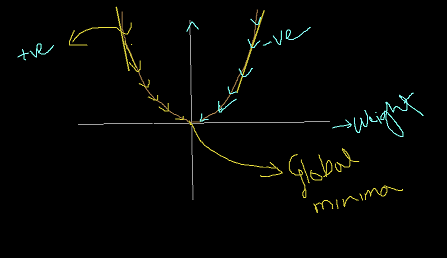# Optimization Techniques In Neural Network

In this article, we are going to discuss some of the popular optimization techniques in Neural Network.

## What is Optimizer in neural network

The optimizer is something by virtue of which we can reduce the loss function of our model(Neural Network). With the help of optimizer, we can change the weight of a neuron, so that the weights can be converged and it can reach to the global minima.

Now the weight optimization function is :   W(new)= W(old)- (a*(dL/dW(old)))
Here a is the learning rate which is basically a very small value. We are just deriving the loss function in terms of the old weight of the neuron.

### What is Gradient Descent: Optimization Technique In Neural Network

Now coming to the part that basically gradient descent is. Gradient Descent is an optimization technique that helps us to converge the weight of the neurons in a particular layer. In this method, we take all the data values at a time and optimize them. This method is straight forward and we can simply put all the data values of a data-frame in the memory. But now we have to understand the deficiency of this approach. Let us assume, there are 1 million samples, so if we try to put them all in the memory then memory cannot support such a large amount of data. So, we have to consider some alternative approaches. Here we can come up with an idea called SGD(Stochastic Gradient Descent) and mini-batch gradient descent.

In the below diagram you can understand how the weights of neurons get decreased through gradient descent and can converge to the global minima.### SGD

In GD we use all the data values at a time but in SGD we use only one data value at a time. Now look carefully if we consider one data value at one epoch, then it will be efficient in terms of memory but not in terms of the time limit. It will take a lot of time to complete the whole training process. So, we came with an approach called Min Batch Gradient Descent.

In mini-batch gradient descent, we will take a fixed amount of data point(obviously less than the total size of the data-frame) and train them in one epoch, in this way in every epochs that fixed the amount of data points will be trained. Suppose , we take K no of data points, then in each interval, we will train those K no of data points. Now it will not give much load on memory and also will not be lagging in terms of the time limit. This K no of data points is called the number of batches in this Optimization technique. Now remember one thing if the total size of the data-frame is N and the number of batches is K, then K will always be less than N (K<N).

Here is the below diagram of how the neural network can converge to global minima for Gradient Descent and also for SGD.Now here is another problem with stochastic gradient descent, that from the above diagram we can see that the weights are get decreased in a zig-zag way for the SGD, but for GD it gets decreased in almost a linear way. The problem with the SGD is that this zig-zag way convergence towards the global minima point creates unnecessary noise which can make further obstruction for our model optimization.

So, to prevent this noise we can come with an idea called SGD with momentum.  Here we will progress with the average weight of the neurons.

So, we have discussed different optimization techniques and their usability in neural network.